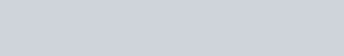# Hypergeometric Distribution: Definition, Properties and Applications

In probability statistics, the hypergeometric distribution is a discrete probability distribution that describes the probability of k successes in n draws, without replacement, from a finite population of size N that contains exactly K objects with that feature, wherein each draw is either a success or a failure.

### Mathematical definition

A discrete random variable X is said to have a  hypergeometric distribution if its probability density function is defined as,If any distribution function is defined by the above probability function then the distribution is called hypergeometric distribution.

where N is a positive integer , M is a non-negative integer that is at most N and n is the positive integer that at most M.

### Properties of Hypergeometric Distribution

• The distribution tends to binomial distribution if N➝∞ and K/N⟶p.
• Hypergeometric distribution is symmetric if p=1/2; positively skewed if p<1/2; negatively skewed if p>1/2.
• The mean of the hypergeometric distribution coincides with the mean of the binomial distribution if M/N=p.

### Application of Hypergeometric Distribution

• The distribution is commonly studied in most introductory probability courses.
• In introducing students to distribution, drawing balls from an urn or selecting playing cards from a deck of cards are often discussed. This is a simple process which focus on sampling without replacement.
• We are also used the distribution to estimate the number of fishes in a lake.
• Election audits typically test a sample of machine-counted precincts to see if recounts by hand or machine match the original counts.

### Hypergeometric test

The hypergeometric test uses the hypergeometric distribution to measure the statistical significance of having drawn a sample consisting of a specific number of k successes (out of n total draws) from a population of size N containing K successes. In a test for over-representation of successes in the sample, the hypergeometric p-value is calculated as the probability of randomly drawing k or more successes from the population in n total draws. In a test for under-representation, the p-value is the probability of randomly drawing k or fewer successes.

The test based on the hypergeometric distribution is identical to the corresponding one-tailed version of Fisher’s exact test. Reciprocally, the p-value of a two-sided Fisher’s exact test can be calculated as the sum of two appropriate hypergeometric tests (for more information see). The test is often used to identify which sub-populations are over- or under-represented in a sample. This test has a wide range of applications. For example, a marketing group could use the test to understand their customer base by testing a set of known customers for over-representation of various demographic subgroups (e.g., women, people under 30).